# Rational approximation with the rSPDE package

## Introduction

In this vignette we will introduce the covariance-based rational SPDE approach and illustrate how to perform statistical inference with it.

The covariance-based approach is an efficient alternative to the operator-based rational SPDE approach by Bolin and Kirchner (2020) which works when one has SPDE driven by Gaussian white noise. We refer the reader to Xiong, Simas, and Bolin (2022) for the theoretical details of the approach.

Details about the operator-based rational SPDE approach are given in the Operator-based rational approximation vignette. For the R-INLA and inlabru implementations of the covariance-based rational SPDE approach we refer the reader to the vignettes R-INLA implementation of the rational SPDE approach and inlabru implementation of the rational SPDE approach respectively.

## Covariance-based rational SPDE approach

Let us first present the basic setup. We want to model the precipitation as a two-dimensional random field $$u$$ within a bounded domain, where each location on the domain associates with a random variable which describe the local precipitation.

In the SPDE approach, introduced in Lindgren, Rue, and Lindström (2011) we model $$u$$ as the solution of the following SPDE: $L^{\alpha/2}(\tau u) = \mathcal{W},$ where $$L = -\Delta +\kappa^2 I$$ and $$\mathcal{W}$$ is the standard Gaussian white noise. Here, $$\alpha$$, $$\kappa$$ and $$\tau$$ are three parameters we want to estimate. In the standard SPDE approach, we write, for a general dimension $$d$$, $$\alpha = \nu + d/2$$ and assume $$\nu$$ to be fixed so that $$\alpha$$ is an integer. In the rational SPDE approach we can use any value of $$\nu>0$$ and also estimate it from data.

Now let us briefly describe how covariance based rational SPDE approach works in statistical inference. The main idea is to perform the rational approximation directly on the covariance operator, which is given by $$L^{-\alpha}$$, instead of on the solution $$u$$. To this end, we begin by obtaining an approximation of the random field $$u$$, which is the solution of the SPDE above, by using the finite element method (FEM): $u_h(\mathbf{s}_i)=\sum_{j=1}^{n_h} \hat{u}_j \varphi_j(\mathbf{s}_i),$ where $$\{\hat{u}_j\}_{j = 1}^{n_h}$$ are stochastic weights and $$\{\varphi_j(\mathbf{s}_i)\}_{j = 1}^{n_h}$$ are fixed piecewise linear and continuous basis functions obtained from a triangulation of the spatial domain. We then obtain a FEM approximation of the operator $$L$$, which is given by $$L_h$$, and the covariance operator of $$u_h$$ is given by $$L_h^{-\alpha}$$.

Now, by using the rational approximation on $$L_h$$, we can approximate covariance operator $$L_h^{-\alpha}$$ as $L_{h,m}^{-\alpha} = L_h^{-\lfloor\alpha\rfloor} p(L_h^{-1})q(L_h^{-1})^{-1},$ where $$\lfloor\alpha\rfloor$$ denotes the integer part of $$\alpha$$, $$m$$ is the order of rational approximation, $$p(L_h^{-1}) = \sum_{i=0}^m a_i L_h^{m-i}$$ and $$q(L_h^{-1}) = \sum_{j=0}^m b_j L_h^{m-i}$$, with $$\{a_i\}_{i = 0}^m$$ and $$\{b_j\}_{j = 0}^m$$ being known coefficients obtained from a rational approximation of the function $$x^{\alpha - \lfloor\alpha\rfloor}$$.

The next step is to perform a partial fraction decomposition of the rational function $$p(L_h^{-1})q(L_h^{-1})^{-1}$$, which yields the representation $L_{h,m}^{-\alpha} =L_h^{-\lfloor\alpha\rfloor} \left(\sum_{i=1}^{m} r_i (L_h-p_i I)^{-1} +k\right).$ Based on the above operator equation, we can write the covariance matrix of the stochastic weights $$\hat{\textbf{u}}$$, where $$\hat{\textbf{u}}=[\hat{u}_1,...,\hat{u}_{n_h}]^\top$$, as $\mathbf{\Sigma}_{\hat{\textbf{u}}} = (\textbf{L}^{-1}\textbf{C})^{\lfloor\alpha\rfloor} \sum_{i=1}^{m}r_i(\textbf{L}-p_i\textbf{C})^{-1}+\textbf{K},$ where $$\textbf{C} = \{C_{ij}\}_{i,j=1}^{n_h}$$, $$C_{ij} = (\varphi_i,\varphi_j)_{L_2(\mathcal{D})}$$, is the mass matrix, $$\textbf{L} = \kappa^2\textbf{C}+\textbf{G}$$, $$\textbf{G} = \{G_{ij}\}_{i,j=1}^{n_h}$$, $$G_{ij}=(\nabla\varphi_i,\nabla\varphi_j)_{L_2(\mathcal{D})}$$, is the stiffness matrix, and $\textbf{K}=\left\{ \begin{array}{lcl} k\textbf{C} & & {\lfloor\alpha\rfloor=0}\\ k\textbf{L}^{-1}(\textbf{C}\textbf{L}^{-1})^{\lfloor\alpha\rfloor-1} & & {\lfloor\alpha\rfloor\geq 1}\\ \end{array} \right. .$

The above representation shows that we can express $$\hat{\textbf{u}}$$ as $\hat{\textbf{u}}=\sum_{k=1}^{m+1}\textbf{x}_k,$ where $$\textbf{x}_k = (x_{k,1}, \ldots, x_{k,n_h})$$, $\textbf{x}_i \sim N(\textbf{0},\textbf{Q}_i^{-1}),$ and $$\textbf{Q}_i$$ is the precision matrix of $$\textbf{x}_i$$, which is given by $\textbf{Q}_i=\left \{ \begin{array}{lcl} (\textbf{L}-p_i\textbf{C})(\textbf{C}^{-1}\textbf{L})^{\lfloor\alpha\rfloor}/r_i, & & {i = 1,...,m}\\ \textbf{K}^{-1}, & & {i = m+1}\\ \end{array}. \right.$

We, then, replace the Matérn latent field by the latent vector given above, which has precision matrix given by $\textbf{Q}=\begin{bmatrix}\textbf{Q}_1& &\\&\ddots&\\& &\textbf{Q}_{m+1}\end{bmatrix}.$ Now, assume we observe $y_i = u_h(\mathbf{s}_i) + \varepsilon_i,\quad i=1,\ldots, N,$ where $$\varepsilon_i\sim N(0,\sigma_\varepsilon^2)$$ are iid measurement noise. Then, we have that $y_i = u_h(\mathbf{s}_i) + \varepsilon_i = \sum_{j=1}^{n_h} \hat{u}_j \varphi_j(\mathbf{s}_i) + \varepsilon_i = \sum_{k=1}^{m+1} \sum_{j=1}^{n_h} x_{k,j} \varphi(\mathbf{s}_i) + \varepsilon_i.$ This can be written in a matrix form as $\textbf{y} = \overline{\textbf{A}} \textbf{X} + \boldsymbol{\varepsilon},$ where $$\textbf{y} = [y_1,\ldots,y_N]^\top, \textbf{X} = [\textbf{x}_1^\top,\ldots,\textbf{x}_{m+1}^\top]^\top$$, $$\boldsymbol{\varepsilon} = [\varepsilon_1,\ldots,\varepsilon_N]^\top$$, $\overline{\textbf{A}}=\begin{bmatrix}\textbf{A}&\cdots&\textbf{A}\end{bmatrix}_{n\times n_h(m+1)},$ and $\textbf{A}=\begin{bmatrix}\varphi_1(s_1)&\cdots&\varphi_{n_h}(s_1)\\\vdots&\vdots&\vdots\\\varphi_1(s_n)&\cdots&\varphi_{n_h}(s_n)\end{bmatrix}.$ We then arrive at the following hierarchical model: \begin{align} \textbf{y}\mid \textbf{X} &\sim N(0,\sigma_\varepsilon\textbf{I})\\ \textbf{X}&\sim N(0,\textbf{Q}^{-1}) \end{align}.

With these elements, we can, for example, use R-INLA to compute the posterior distribution of the three parameters we want to estimate.

## Constructing the approximation

In this section, we explain how to to use the function matern.operators() with the default argument type, that is, type="covariance", which is constructs the covariance-based rational approximation. We will also illustrate the usage of several methods and functions related to the covariance-based rational approximation. We will use functions to sample from Gaussian fields with stationary Matérn covariance function, compute the log-likelihood function, and do spatial prediction.

The first step for performing the covariance-based rational SPDE approximation is to define the FEM mesh. For illustration purposes, the rSPDE package contains a simple FEM implementation for models on $$\mathbb{R}$$ which we will use first. We will also illustrate how spatial models can be constructed if the FEM implementation of the R-INLA package is used instead. When using the R-INLA package, we also recommend the usage of our R-INLA implementation of the rational SPDE approach. For more details, see the R-INLA implementation of the rational SPDE approach vignette.

We begin by loading the rSPDE package:

library(rSPDE)

Assume that we want to define a model on the interval $$[0,1]$$. We then start by defining a vector with mesh nodes $$s_i$$ where the basis functions $$\varphi_i$$ are centered.

s <- seq(from = 0, to = 1, length.out = 101)

Based on these nodes, we use the built-in function rSPDE.fem1d() to assemble two matrices needed for creating the approximation of a basic Matérn model. These matrices are the mass matrix $$\boldsymbol{\mathrm{C}}$$, with elements $$C_{ij} = \int \varphi_j(s) \varphi_i(s) ds$$, and the stiffness matrix $$\boldsymbol{\mathrm{G}}$$, with elements $$G_{ij} = \int \nabla\varphi_j(s) \cdot \nabla\varphi_i(s) ds$$.

fem <- rSPDE.fem1d(s)

We can now use matern.operators() to construct a rational SPDE approximation of order $$m=2$$ for a Gaussian random field with a Matérn covariance function on the interval. We also refer the reader to the Operator-based rational approximation for a similar comparison made for the operator-based rational approximation.

kappa <- 20
sigma <- 2
nu <- 0.8
op_cov <- matern.operators(
C = fem$C, G = fem$G, nu = nu,
kappa = kappa, sigma = sigma, d = 1, m = 2
)

The object op_cov contains the matrices needed for evaluating the distribution of the stochastic weights $$\boldsymbol{\mathrm{u}}$$. If we want to evaluate $$u_h(s)$$ at some locations $$s_1,\ldots, s_n$$, we need to multiply the weights with the basis functions $$\varphi_i(s)$$ evaluated at the locations. For this, we can construct the observation matrix $$\boldsymbol{\mathrm{A}}$$, with elements $$A_{ij} = \varphi_j(s_i)$$, which links the FEM basis functions to the locations. This matrix can be constructed using the function rSPDE.A1d(). However, as observed in the introduction of this vignette, we have decomposed the stochastic weights $$\boldsymbol{\mathrm{u}}$$ into a vector of latent variables. Thus, the $$A$$ matrix for the covariance-based rational approximation, which we will denote by $$\overline{A}$$, is actually given by the $$m+1$$-fold horizontal concatenation of these $$A$$ matrices, where $$m$$ is the order of the rational approximation.

To compute the precision matrix from the covariance-based rational approximation one can use the precision() method on the CBrSPDEobj object (the object returned by the matern.operators() function with the default type, which is type="covariance"):

Q <- precision(op_cov)

To evaluate the accuracy of the approximation, let us compute the covariance function between the process at $$s=0.5$$ and all other locations in s and compare with the true Matérn covariance function. The covariances can be calculated as $\overline{\boldsymbol{\mathrm{A}}} \boldsymbol{\mathrm{Q}}^{-1}\overline{\boldsymbol{\mathrm{v}}}.$ Here, $$\boldsymbol{\mathrm{Q}}$$ is the precision matrix obtained from the covariance-based rational approximation, $$\boldsymbol{\mathrm{A}}$$ is an identity matrix since we are evaluating the approximation in the nodes of the FEM mesh, $$\overline{\boldsymbol{\mathrm{v}}}$$ is the $$(m+1)$$-fold vertical concatenation of the vector $$\boldsymbol{\mathrm{v}}$$, where $$\boldsymbol{\mathrm{v}}$$ is a vector with all basis functions evaluated in $$s=0.5$$.

v <- t(rSPDE.A1d(s, 0.5))
v_bar <- kronecker(matrix(1, nrow = 3), v)
A <- Diagonal(101)
A_bar <- kronecker(matrix(1, ncol = 3), A)
c_cov.approx <- (A_bar) %*% solve(Q, v_bar)

Let us now compute the true Matérn covariance function on the interval $$(0,1)$$, which is the folded Matérn, see Theorem 1 in An explicit link between Gaussian fields and Gaussian Markov random fields: the stochastic partial differential equation approach for further details.

c.true <- folded.matern.covariance.1d(rep(0.5, length(s)), abs(s), kappa, nu, sigma)

The covariance function and the error compared with the Matérn covariance are shown in the following figure.

opar <- par(
mfrow = c(1, 2), mgp = c(1.3, 0.5, 0),
mar = c(2, 2, 0.5, 0.5) + 0.1
)
plot(s, c.true,
type = "l", ylab = "C(|s-0.5|)", xlab = "s", ylim = c(0, 5),
cex.main = 0.8, cex.axis = 0.8, cex.lab = 0.8
)
lines(s, c_cov.approx, col = 2)
legend("topright",
bty = "n",
legend = c("Matérn", "Rational"),
col = c("black", "red"),
lty = rep(1, 2), ncol = 1,
cex = 0.8
)

plot(s, c.true - c_cov.approx,
type = "l", ylab = "Error", xlab = "s",
cex.main = 0.8, cex.axis = 0.8, cex.lab = 0.8
)
par(opar)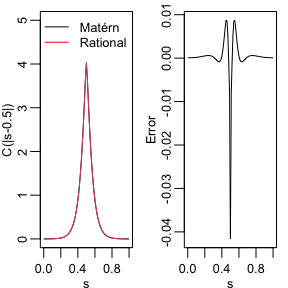To improve the approximation we can increase the degree of the polynomials, by increasing $$m$$, and/or increase the number of basis functions used for the FEM approximation. Let us, for example, compute the approximation with $$m=4$$ using the same mesh, as well as the approximation when we increase the number of basis functions and use $$m=2$$ and $$m=4$$.

op_cov2 <- matern.operators(
kappa = kappa, sigma = sigma, nu = nu,
G = fem$G, C = fem$C, d = 1, m = 4
)
Q2 <- precision(op_cov2)
v_bar2 <- kronecker(matrix(1, nrow = 5), v)
A_bar2 <- kronecker(matrix(1, ncol = 5), A)
c_cov.approx2 <- (A_bar2) %*% solve(Q2, v_bar2)

s2 <- seq(from = 0, to = 1, length.out = 501)
fem2 <- rSPDE.fem1d(s2)
op_cov <- matern.operators(
kappa = kappa, sigma = sigma, nu = nu,
G = fem2$G, C = fem2$C, d = 1, m = 2
)
Q3 <- precision(op_cov)
A2 <- rSPDE.A1d(s2, s)
v2 <- t(rSPDE.A1d(s2, 0.5))
v2_bar <- kronecker(matrix(1, nrow = 3), v2)
A2_bar <- kronecker(matrix(1, ncol = 3), A2)
c_cov.approx3 <- (A2_bar) %*% solve(Q3, v2_bar)

op_cov <- matern.operators(
kappa = kappa, sigma = sigma, nu = nu,
G = fem2$G, C = fem2$C, d = 1, m = 4
)
Q4 <- precision(op_cov)
v2_bar2 <- kronecker(matrix(1, nrow = 5), v2)
A2_bar2 <- kronecker(matrix(1, ncol = 5), A2)
c_cov.approx4 <- (A2_bar2) %*% solve(Q4, v2_bar2)

The resulting errors are shown in the following figure.

opar <- par(mgp = c(1.3, 0.5, 0), mar = c(2, 2, 0.5, 0.5) + 0.1)
plot(s, c.true - c_cov.approx,
type = "l", ylab = "Error", xlab = "s", col = 1,
cex.main = 0.8, cex.axis = 0.8, cex.lab = 0.8
)
lines(s, c.true - c_cov.approx2, col = 2)
lines(s, c.true - c_cov.approx3, col = 3)
lines(s, c.true - c_cov.approx4, col = 4)
legend("bottomright",
bty = "n",
legend = c("m=2 coarse mesh", "m=4 coarse mesh", "m=2 fine mesh", "m=4 fine mesh"),
col = c(1, 2, 3, 4),
lty = rep(1, 2), ncol = 1,
cex = 0.8
)
par(opar)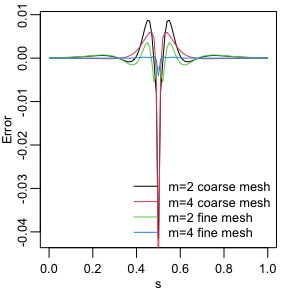Since the error induced by the rational approximation decreases exponentially in $$m$$, there is in general rarely a need for an approximation with a large value of $$m$$. This is good because the size of $$\boldsymbol{\mathrm{Q}}$$ increases with $$m$$, which makes the approximation more computationally costly to use. To illustrate this, let us compute the norm of the approximation error for different $$m$$.

errors <- rep(0, 4)
for (i in 1:4) {
op_cov <- matern.operators(
kappa = kappa, sigma = sigma, nu = nu,
G = fem2$G, C = fem2$C, d = 1, m = i
)
Q <- precision(op_cov)
v_bar <- kronecker(matrix(1, nrow = i + 1), v2)
A_bar <- kronecker(matrix(1, ncol = i + 1), A2)
c_cov.approx <- (A_bar) %*% solve(Q, v_bar)
errors[i] <- norm(c.true - c_cov.approx)
}
print(errors)
##  0.977500618 0.086659189 0.017335545 0.008432139

We see that the error decreases very fast when we increase $$m$$ from $$1$$ to $$4$$, without any numerical instability. This is an advantage of the covariance-based rational approximation when compared to the operator-based rational approximation. See Operator-based rational approximation for details on the numerical instability of the operator-based rational approximation.

## Using the approximation

When we use the function matern.operators(), we can simulate from the model using the simulate() method. To such an end we simply apply the simulate() method to the object returned by the matern.operators() function:

u <- simulate(op_cov)

If we want replicates, we simply set the argument nsim to the desired number of replicates. For instance, to generate two replicates of the model, we simply do:

u.rep <- simulate(op_cov, nsim = 2)

### Fitting a model

There is built-in support for computing log-likelihood functions and performing kriging prediction in the rSPDE package. To illustrate this, we use the simulation to create some noisy observations of the process. For this, we first construct the observation matrix linking the FEM basis functions to the locations where we want to simulate. We first randomly generate some observation locations and then construct the matrix.

set.seed(1)
s <- seq(from = 0, to = 1, length.out = 501)
n.obs <- 200
obs.loc <- runif(n.obs)
fem <- rSPDE.fem1d(s)
A <- rSPDE.A1d(s, obs.loc)

We now generate the observations as $$Y_i = u(s_i) + \varepsilon_i$$, where $$\varepsilon_i \sim N(0,\sigma_e^2)$$ is Gaussian measurement noise. We will assume that the latent process has a Matérn covariance with $$\kappa=20, \sigma=2$$ and $$\nu=0.8$$:

kappa <- 20
sigma <- 2
nu <- 0.8
op_cov <- matern.operators(
C = fem$C, G = fem$G, nu = nu,
kappa = kappa, sigma = sigma, d = 1, m = 2
)
tau <- op_cov$tau u <- simulate(op_cov) sigma.e <- 0.3 Y <- as.vector(A %*% u + sigma.e * rnorm(n.obs)) Let us now fit the model. To this end we first must compute the loglikelihood function as function of the parameters we want to estimate. We define the loglikelihood function parametrized using the logarithm of each parameter to avoid constrained optimization. mlik_cov <- rSPDE.construct.matern.loglike(op_cov, Y=Y, A=A) We will now get suitable initial values for the optimization using the get.initial.values.rSPDE(). We then add an initial guess for sigma.e. theta0 <- c( get.initial.values.rSPDE(mesh.range = 1, dim = 1, parameterization = "spde"), log(0.1 * sqrt(var(as.vector(Y)))) ) start_time <- Sys.time() theta <- optim(theta0, mlik_cov, method = "L-BFGS-B" ) end_time <- Sys.time() time_optim <- end_time - start_time print(data.frame( tau = c(tau, exp(theta$par)), kappa = c(kappa, exp(theta$par)), nu = c(nu, exp(theta$par)), sigma.e = c(sigma.e, exp(theta$par)), row.names = c("Truth", "Estimates") )) ## tau kappa nu sigma.e ## Truth 0.02753295 20.00000 0.8000000 0.3000000 ## Estimates 0.02283270 18.00965 0.8176277 0.3302515 # Total time print(time_optim) ## Time difference of 17.36191 secs We can also speed up the optimization by using the optimParallel() function from the optimParallel package. To such an end, we simply replace the optim() function by optimParallel() and set the number of cores we want to use: library(optimParallel) # Preparing the parallel # Checking if we have a limit to the number of cores chk <- Sys.getenv("_R_CHECK_LIMIT_CORES_", "") if (nzchar(chk) && chk == "TRUE") { n_cores <- 2L } else { n_cores <- parallel::detectCores() - 1 } cl <- makeCluster(n_cores) setDefaultCluster(cl = cl) # Exporting the needed objects to the parallel cores # This step is not necessary for the regular optim parallel::clusterExport(cl, "op_cov") parallel::clusterExport(cl, "Y") parallel::clusterExport(cl, "A") start_time <- Sys.time() theta_parallel <- optimParallel(theta0, mlik_cov) end_time <- Sys.time() time_optim <- end_time - start_time print(data.frame( tau = c(tau, exp(theta_parallel$par)),
kappa = c(kappa, exp(theta_parallel$par)), nu = c(nu, exp(theta_parallel$par)),
sigma.e = c(sigma.e, exp(theta_parallel$par)), row.names = c("Truth", "Estimates") )) ## tau kappa nu sigma.e ## Truth 0.02753295 20.00000 0.8000000 0.3000000 ## Estimates 0.02283270 18.00965 0.8176277 0.3302515 # Total time print(time_optim) ## Time difference of 13.19101 secs ### Kriging Finally, we compute the kriging prediction of the process $$u$$ at the locations in s based on these observations. To specify which locations that should be predicted, the argument Aprd is used. This argument should be an observation matrix that links the mesh locations to the prediction locations. A.krig <- rSPDE.A1d(s, s) Let us update the CBrSPDEobj object (returned by the matern.operators() function) with the fitted parameters: tau_est <- exp(theta$par)
kappa_est <- exp(theta$par) nu_est <- exp(theta$par)

op_cov <- update(op_cov,
user_kappa = kappa_est,
user_tau = tau_est,
user_nu = nu_est
)

We can now perform kriging with the predict() method:

u.krig <- predict(op_cov, A = A, Aprd = A.krig, Y = Y, sigma.e = sigma.e)

The simulated process, the observed data, and the kriging prediction are shown in the following figure.

opar <- par(mgp = c(1.3, 0.5, 0), mar = c(2, 2, 0.5, 0.5) + 0.1)
plot(obs.loc, Y,
ylab = "u(s)", xlab = "s",
ylim = c(min(c(min(u), min(Y))), max(c(max(u), max(Y)))),
cex.main = 0.8, cex.axis = 0.8, cex.lab = 0.8
)
lines(s, u)
lines(s, u.krig$mean, col = 2) par(opar)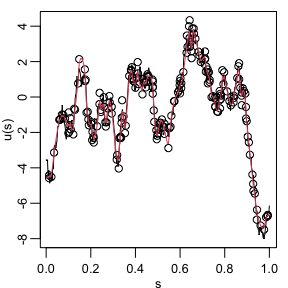## Fitting a model with replicates Let us illustrate how to simulate a dataset with replicates and then fit a model to such data. Recall that to simulate a latent model with replicates, all we do is set the nsim argument to the number of replicates. We will use the CBrSPDEobj object (returned from the matern.operators() function) from the previous example, namely op_cov. set.seed(123) n.rep <- 20 u.rep <- simulate(op_cov, nsim = n.rep) Now, let us generate the observed values $$Y$$: sigma.e <- 0.3 Y.rep <- A %*% u.rep + sigma.e * matrix(rnorm(n.obs * n.rep), ncol = n.rep) Note that $$Y$$ is a matrix with 20 columns, each column containing one replicate. Now, the remaining of the code is identical to the previous case. The rSPDE.matern.loglike() function automatically identifies the replicates from the fact that $$Y$$ is a matrix with more than one column. theta0 <- c( get.initial.values.rSPDE(mesh.range = 1, dim = 1, parameterization = "spde"), log(0.1 * sqrt(var(as.vector(Y)))) ) mlik_cov <- rSPDE.construct.matern.loglike(op_cov, Y=Y.rep, A=A) # Exporting the needed objects to the parallel cores # This step is not necessary for the regular optim parallel::clusterExport(cl, "op_cov") parallel::clusterExport(cl, "Y.rep") parallel::clusterExport(cl, "A") start_time <- Sys.time() theta <- optimParallel(theta0, mlik_cov, method = "L-BFGS-B" ) end_time <- Sys.time() time_optim <- end_time - start_time print(data.frame( tau = c(tau, exp(theta$par)), kappa = c(kappa, exp(theta$par)), nu = c(nu, exp(theta$par)), sigma.e = c(sigma.e, exp(thetapar)), row.names = c("Truth", "Estimates") )) ## tau kappa nu sigma.e ## Truth 0.02753295 20.0000 0.800000 0.3000000 ## Estimates 0.02719025 17.3714 0.784712 0.2932504 # Total time print(time_optim) ## Time difference of 11.8331 secs ## Spatial data and parameter estimation The functions used in the previous examples also work for spatial models. We then need to construct a mesh over the domain of interest and then compute the matrices needed to define the operator. These tasks can be performed, for example, using the R-INLA package. Let us start by defining a mesh over $$[0,1]\times [0, 1]$$ and compute the mass and stiffness matrices for that mesh. It is important to mention that when using the R-INLA package we recommend the usage of the R-INLA implementation of the rational SPDE approach. The purpose of this section is to show that we can estimate the model without R-INLA and using a maximum likelihood approach instead. We consider a simple Gaussian linear model with 30 independent replicates of a latent spatial field $$u(\mathbf{s})$$, observed at the same $$m$$ locations, $$\{\mathbf{s}_1 , \ldots , \mathbf{s}_m \}$$, for each replicate. For each $$i = 1,\ldots,m,$$ we have \begin{align} y_i &= u_1(\mathbf{s}_i)+\varepsilon_i,\\ \vdots &= \vdots\\ y_{i+29m} &= u_{30}(\mathbf{s}_i) + \varepsilon_{i+29m}, \end{align} where $$\varepsilon_1,\ldots,\varepsilon_{30m}$$ are iid normally distributed with mean 0 and standard deviation 0.1. Let us create the FEM mesh: library(INLA) n_loc <- 500 loc_2d_mesh <- matrix(runif(n_loc * 2), n_loc, 2) mesh_2d <- inla.mesh.2d( loc = loc_2d_mesh, cutoff = 0.05, offset = c(0.1, 0.4), max.edge = c(0.05, 0.5) ) plot(mesh_2d, main = "") points(loc_2d_mesh[, 1], loc_2d_mesh[, 2])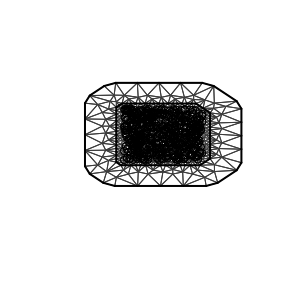We can now use this mesh to define a rational SPDE approximation of order $$m=2$$ for a Matérn model in the same fashion as we did above in the one-dimensional case. We now simulate a latent process with standard deviation $$\sigma=1$$ and range $$0.1$$. We will use $$\nu=0.5$$ so that the model has an exponential covariance function. To this end we create a model object with the matern.operators() function: nu <- 0.5 sigma <- 1 range <- 0.1 kappa <- sqrt(8 * nu) / range d <- 2 op_cov_2d <- matern.operators( mesh = mesh_2d, nu = nu, kappa = kappa, sigma = sigma, m = 2 ) tau <- op_cov_2dtau

Now let us simulate some noisy data that we will use to estimate the parameters of the model. To construct the observation matrix, we use the R-INLA function inla.spde.make.A(). Recall that we will simulate the data with 30 replicates.

n.rep <- 30
u <- simulate(op_cov_2d, nsim = n.rep)
A <- inla.spde.make.A(
mesh = mesh_2d,
loc = loc_2d_mesh
)
sigma.e <- 0.1
Y <- A %*% u + matrix(rnorm(n_loc * n.rep), ncol = n.rep) * sigma.e

The first replicate of the simulated random field as well as the observation locations are shown in the following figure.

library(viridis)
library(ggplot2)
proj <- inla.mesh.projector(mesh_2d, dims = c(70, 70))

df_field <- data.frame(x = proj$lattice$loc[,1],
y = proj$lattice$loc[,2],
field = as.vector(inla.mesh.project(proj,
field = as.vector(u[, 1]))),
type = "field")

df_loc <- data.frame(x = loc_2d_mesh[, 1],
y = loc_2d_mesh[, 2],
field = as.vector(Y[,1]),
type = "locations")
df_plot <- rbind(df_field, df_loc)

ggplot(df_plot) + aes(x = x, y = y, fill = field) +
facet_wrap(~type) + xlim(0,1) + ylim(0,1) +
geom_raster(data = df_field) +
geom_point(data = df_loc, aes(colour = field),
show.legend = FALSE) +
scale_fill_viridis() + scale_colour_viridis()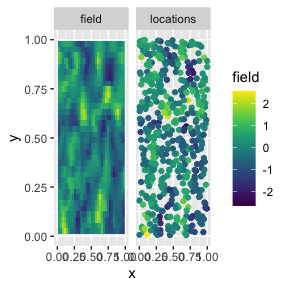We now use the function rSPDE.matern.loglike() to define the likelihood. This function is object-based, in the sense that it obtains several of the quantities it needs from the rSPDE model object. In our case, for this example, the object op_cov_2d.

To simplify parameter estimation, we create an objective function to minimize which is the negative log-likelihood, parametrized using the logarithm of each parameter to avoid constrained optimization.

mlik_2d <- rSPDE.construct.matern.loglike(op_cov_2d, Y=Y, A=A)

We can now estimate the parameter using optimParallel():

theta0_2d <- c(
get.initial.values.rSPDE(mesh = mesh_2d,
parameterization = "spde"),
log(0.1 * sqrt(var(as.vector(Y))))
)

# Exporting the needed objects to the parallel cores
# This step is not necessary for the regular optim
parallel::clusterExport(cl, "op_cov_2d")
parallel::clusterExport(cl, "Y")
parallel::clusterExport(cl, "A")

start_time <- Sys.time()
pars <- optimParallel(theta0_2d, mlik_2d)
end_time <- Sys.time()
total_time <- end_time - start_time
results <- data.frame(
tau = c(tau, exp(pars$par)), kappa = c(kappa, exp(pars$par)),
nu = c(nu, exp(pars$par)), sigma.e = c(sigma.e, exp(pars$par)),
row.names = c("True", "Estimate")
)
print(results)
##                 tau    kappa        nu   sigma.e
## True     0.08920621 20.00000 0.5000000 0.1000000
## Estimate 0.05036810 22.72679 0.6382482 0.1004502
# Total time
print(total_time)
## Time difference of 18.22598 secs

## An example with a non-stationary model

Our goal now is to show how one can fit model with non-stationary $$\sigma$$ (std. deviation) and non-stationary $$\rho$$ (a range parameter). One can also use the parameterization in terms of non-stationary SPDE parameters $$\kappa$$ and $$\tau$$.

For this example we will consider simulated data.

### Simulating the data

Let us consider a simple Gaussian linear model with a latent spatial field $$x(\mathbf{s})$$, defined on the rectangle $$(0,10) \times (0,5)$$, where the std. deviation and range parameter satisfy the following log-linear regressions: \begin{align} \log(\sigma(\mathbf{s})) &= \theta_1 + \theta_3 b(\mathbf{s}),\\ \log(\rho(\mathbf{s})) &= \theta_2 + \theta_3 b(\mathbf{s}), \end{align} where $$b(\mathbf{s}) = (s_1-5)/10$$. We assume the data is observed at $$m$$ locations, $$\{\mathbf{s}_1 , \ldots , \mathbf{s}_m \}$$. For each $$i = 1,\ldots,m,$$ we have

$y_i = x_1(\mathbf{s}_i)+\varepsilon_i,$

where $$\varepsilon_1,\ldots,\varepsilon_{m}$$ are iid normally distributed with mean 0 and standard deviation 0.1.

We begin by defining the domain and creating the mesh:

rec_domain <- cbind(c(0, 1, 1, 0, 0) * 10, c(0, 0, 1, 1, 0) * 5)

mesh <- inla.mesh.2d(loc.domain = rec_domain, cutoff = 0.1,
max.edge = c(0.5, 1.5), offset = c(0.5, 1.5))

We follow the same structure as INLA. However, INLA only allows one to specify B.tau and B.kappa matrices, and, in INLA, if one wants to parameterize in terms of range and standard deviation one needs to do it manually. Here we provide the option to directly provide the matrices B.sigma and B.range.

The usage of the matrices B.tau and B.kappa are identical to the corresponding ones in inla.spde2.matern() function. The matrices B.sigma and B.range work in the same way, but they parameterize the stardard deviation and range, respectively.

The columns of the B matrices correspond to the same parameter. The first column does not have any parameter to be estimated, it is a constant column.

So, for instance, if one wants to share a parameter with both sigma and range (or with both tau and kappa), one simply let the corresponding column to be nonzero on both B.sigma and B.range (or on B.tau and B.kappa).

We will assume $$\nu = 0.8$$, $$\theta_1 = 0, \theta_2 = 1$$ and $$\theta_3=1$$. Let us now build the model with the spde.matern.operators() function:

nu <- 0.8
true_theta <- c(0,1, 1)
B.sigma = cbind(0, 1, 0, (mesh$loc[,1] - 5) / 10) B.range = cbind(0, 0, 1, (mesh$loc[,1] - 5) / 10)

# SPDE model
op_cov_ns <- spde.matern.operators(mesh = mesh,
theta = true_theta,
nu = nu,
B.sigma = B.sigma,
B.range = B.range)

Let us now sample the data with the simulate() method:

u <- as.vector(simulate(op_cov_ns, seed = 123))

Let us now obtain 600 random locations on the rectangle and compute the $$A$$ matrix:

m <-600
loc_mesh <- cbind(runif(m) * 10, runif(m) * 5)

A <- inla.spde.make.A(
mesh = mesh,
loc = loc_mesh
)

We can now generate the response vector y:

y <- as.vector(A %*% as.vector(u)) + rnorm(m) * 0.1

### Fitting the non-stationary rSPDE model

We can build the likelihood function using the function factory construct.spde.matern.loglike():

mlik <- construct.spde.matern.loglike(op_cov_ns, y, A)

Let us now choose some reasonable starting values (on log scale) depending on the size of the domain with the get.initial.values.rSPDE() function:

theta0 <- c(get.initial.values.rSPDE(mesh = mesh, B.sigma = B.sigma, B.range = B.range), log(0.01))

Let us now fit the model:

theta <- optim(theta0, mlik)

Let us now compare with the true values:

print(data.frame(
theta1 = c(true_theta, theta$par), theta2 = c(true_theta, theta$par),
theta3 = c(true_theta, theta$par), nu = c(nu, exp(theta$par)), sigma.e = c(sigma.e, exp(theta$par)), row.names = c("Truth", "Estimates") )) ## theta1 theta2 theta3 nu sigma.e ## Truth 0.00000000 1.0000000 1.000000 0.8000000 0.1000000 ## Estimates 0.06419504 0.8512005 1.297721 0.9054998 0.1039849 ## Changing the type and the order of the rational approximation We have three rational approximations available. The BRASIL algorithm Hofreither (2021), and two “versions” of the Clenshaw-Lord Chebyshev-Pade algorithm, one with lower bound zero and another with the lower bound given in Xiong, Simas, and Bolin (2022). The type of rational approximation can be chosen by setting the type_rational_approximation argument in the matern.operators function. The BRASIL algorithm corresponds to the choice brasil, the Clenshaw-Lord Chebyshev pade with zero lower bound and non-zero lower bounds are given, respectively, by the choices chebfun and chebfunLB. For instance, we can create an rSPDE object with a chebfunLB rational approximation by op_cov_2d_type <- matern.operators( mesh = mesh_2d, nu = nu, kappa = kappa, sigma = sigma, m = 2, type_rational_approximation = "chebfunLB" ) tau <- op_cov_2d_type$tau

We can check the order of the rational approximation with the rational.order() function and assign a new order with the rational.order<-() function:

rational.order(op_cov_2d_type)
##  2
rational.order(op_cov_2d_type) <- 3

Let us fit a model using the data from the previous example:

mlik_2d_type <- rSPDE.construct.matern.loglike(op_cov_2d_type, Y=Y, A=A)

We can now estimate the parameter using optimParallel():

# Exporting the needed objects to the parallel cores
# This step is not necessary for the regular optim
parallel::clusterExport(cl, "op_cov_2d_type")

start_time <- Sys.time()
pars <- optimParallel(theta0_2d, mlik_2d_type)
end_time <- Sys.time()
total_time <- end_time - start_time
results <- data.frame(
tau = c(tau, exp(pars$par)), kappa = c(kappa, exp(pars$par)),
nu = c(nu, exp(pars$par)), sigma.e = c(sigma.e, exp(pars$par)),
row.names = c("True", "Estimate")
)
print(results)
##                 tau    kappa        nu   sigma.e
## True     0.02870953 20.00000 0.8000000 0.1000000
## Estimate 0.05720811 22.23146 0.6068955 0.1004481
# Total time
print(total_time)
## Time difference of 56.33814 secs

Finally, we can check the type of rational approximation with the rational.type() function and assign a new type by using the rational.type<-() function:

rational.type(op_cov_2d_type)
##  "chebfunLB"
rational.type(op_cov_2d_type) <- "brasil"

Let us now fit this model, with the data from the previous example, with brasil rational approximation:

mlik_2d_type <- rSPDE.construct.matern.loglike(op_cov_2d_type, Y=Y, A=A)

We can now estimate the parameter using optimParallel():

# Exporting the needed objects to the parallel cores
# This step is not necessary for the regular optim
parallel::clusterExport(cl, "op_cov_2d_type")

start_time <- Sys.time()
pars <- optimParallel(theta0_2d, mlik_2d_type)
end_time <- Sys.time()
total_time <- end_time - start_time
results <- data.frame(
tau = c(tau, exp(pars$par)), kappa = c(kappa, exp(pars$par)),
nu = c(nu, exp(pars$par)), sigma.e = c(sigma.e, exp(pars$par)),
row.names = c("True", "Estimate")
)
print(results)
##                 tau    kappa        nu   sigma.e
## True     0.02870953 20.00000 0.8000000 0.1000000
## Estimate 0.06358789 21.85246 0.5804641 0.1004512
# Total time
print(total_time)
## Time difference of 25.27517 secs